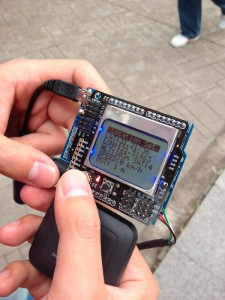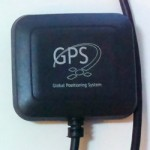After modifying a USB GPS receiver to use with Arduino, I continued to make a further attempt to measure distance between two locations with Arduino. The idea is straight-forward, when pressing a key, current location is stored and used as start location. The line distance of current location and the start location is calculated and displayed on the LCD on the spot. Next steps are: (1) allowing entering GPS coordinates of a location (2) calculating and displaying trip distance.

If the distance between two locations is not too long, the calculation of the line distance between them can be simplified a lot by treating the earth as flat.

For example:

The longitude of location A is 116.95400 and the latitude of it is 39.95400.
The longitude of location B is 116.95300 and the latitude of it is 39.95300.
The distance between A and B is rounded to:
[ (11695400 – 11695300)^2 + (3995400 – 3995300)^2 ] ^(1/2) =(10000+10000) ^ (1/2) =141 (meter)

My sketch:

/********************************************************************
* Arduino GPS Distance Calculator
********************************************************************/

#include <arduino.h>
#include <TinyGPS.h>
#include <LCD4884.h>
#include <math.h>

#define DEBOUNCE_MAX 15
#define DEBOUNCE_ON  10
#define DEBOUNCE_OFF 3

#define NUM_KEYS 5
// joystick number
#define KEY_LEFT 0
#define KEY_CENTER 1
#define KEY_DOWN 2
#define KEY_RIGHT 3
#define KEY_UP 4

50, 200, 400, 600, 800 };

// debounce counters
byte button_count[NUM_KEYS];
// button status - pressed/released
byte button_status[NUM_KEYS];
// button on flags for user program
byte button_flag[NUM_KEYS];

// Convert ADC value to key number
char get_key(unsigned int input)
{
char k;
for (k = 0; k < NUM_KEYS; k++) {
return k;
}
}

if (k >= NUM_KEYS)
k = -1;     // No valid key pressed

return k;
}

for(byte i=0; i<NUM_KEYS; i++) {
if(key_in==i) {
//one key is pressed
if(button_count[i]<DEBOUNCE_MAX) {
button_count[i]++;
if(button_count[i]>DEBOUNCE_ON) {
if(button_status[i] == 0) {
button_flag[i] = 1;
button_status[i] = 1; //button debounced to 'pressed' status
}
}
}
} else {
// no button pressed
if (button_count[i] >0) {
button_flag[i] = 0;
button_count[i]--;
if(button_count[i]<DEBOUNCE_OFF) {
button_status[i]=0;   //button debounced to 'released' status
}
}
}

}
}

TinyGPS gps;

byte CheckPressedKey()
{
for(int i=0; i<NUM_KEYS; i++){
if(button_flag[i] !=0){
button_flag[i]=0;  // reset button flag
return i;
}
}
return -1;
}

void setup()
{
// reset button arrays
for(byte i=0; i<NUM_KEYS; i++){
button_count[i]=0;
button_status[i]=0;
button_flag[i]=0;
}

// Setup timer2 -- Prescaler/256
TCCR2A &= ~((1<<WGM21) | (1<<WGM20));
TCCR2B &= ~(1<<WGM22);
TCCR2B = (1<<CS22)|(1<<CS21);

ASSR |=(0<<AS2);

// Use normal mode
TCCR2A =0;
//Timer2 Overflow Interrupt Enable
TIMSK2 |= (0<<OCIE2A);
TCNT2=0x6;  // counting starts from 6;
TIMSK2 = (1<<TOIE2);

SREG|=1<<SREG_I;

lcd.LCD_init();
lcd.LCD_clear();
lcd.LCD_write_string(0, 0, " WAITING GPS  ", MENU_HIGHLIGHT);

Serial.begin(9600);
delay(2000);
}

void loop()
{
static unsigned long lastTime = 0;
static long originLat = 0;
static long originLon = 0;
static long lat = 0;
static long lon = 0;

switch (CheckPressedKey()) {
case KEY_CENTER:
originLat = lat;
originLon = lon;
lcd.LCD_write_string(0, 0, " Location Set ", MENU_HIGHLIGHT);
delay(1000);
break;
case KEY_DOWN:
lcd.backlight(0);
break;
case KEY_UP:
lcd.backlight(1);
break;
}

if (!Serial.available()) return;

// push NMEA characters to TinyGPS
if (!gps.encode(c)) {
lcd.LCD_putchar(c);
}
return;
}

// update screen every 500ms
if (millis() - lastTime > 500) {
char buf;
unsigned long fix_age;
unsigned long speed;
unsigned long date, time;

// put up the ready sign and clear the screen
lcd.LCD_clear();
}

gps.get_datetime(&date, &time, &fix_age);
sprintf(buf, "%06ld %08ld", date, time);

gps.get_position(&lat, &lon, &fix_age);
sprintf(buf, "LAT:%d.%05ld",
(int)(lat / 100000), lat % 100000);
sprintf(buf, "LON:%d.%05ld",
(int)(lon / 100000), lon % 100000);
sprintf(buf, "ALT:%dm SAT:%d ",
(int)(gps.altitude() / 100), gps.satellites());

speed = gps.speed();
if (speed > 1000) speed = 0;
// 1knot = 1.852km
sprintf(buf, "SPEED:%d km/h  ", (int)(speed * 1852 / 100000));

// distance
if (originLat != 0) {
long distance = sqrt((lat - originLat) * (lat - originLat) + (lon - originLon) * (lon - originLon));
sprintf(buf, "DST: %ld m    ", distance);
}

lastTime = millis();
}
}

// Timer2 interrupt routine -
// 1/(160000000/256/(256-6)) = 4ms interval
ISR(TIMER2_OVF_vect) {
TCNT2  = 6;
}

If you need a GPS receiver for Arduino, check this out:• Low-power consumption SIRF 3 chip
• No breakout needed for use with Arduino
• 9600Hz TTL UART interface
• Working with Arduino and TinyGPS
• Built-in antenna and mounting magnet
• Size:52x48x10 mm
• Weight: 80g
• Price: US\$ 35 (free shipping worldwide)